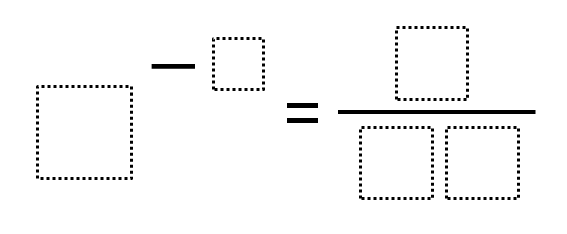# Negative Exponents – Closest to Zero

Directions: Using the digits 0 to 9, at most one time each, fill in the boxes to make a result that is as close to zero as possible.### Hint

What happens when you have a negative exponent?
Where does the 1 go?

8 ^ (-2) = 1 / 64

Source: Daniel Luevanos

## Systems of Equations 1

Directions: Using the integers -9 to 9 at most one time each, fill in the …

1.How can you use the 4 in two places in the equation? If that’s allowed, then 2 ^ -6 = 1/64 should also be allowed.

•Or 9^-2 = 1/81
or 3^-4 = 1/81

•Good spot Ms. M. I have removed that solution.

2.So, it seems 7^-2 = 1/49 and 8^-2 = 1/64 are the only solutions which use unique digits, with the latter being closer to zero.